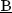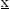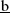# A quantum algorithm for spin chemistry: a Bayesian exchange coupling parameter calculator with broken-symmetry wave functions.

The Heisenberg exchange coupling parameter J (H = −2JSi · Sj) characterises the isotropic magnetic interaction between unpaired electrons, and it is one of the most important spin Hamiltonian parameters of multi-spin open shell systems. The J value is related to the energy difference between high-spin and low-spin states, and thus computing the energies of individual spin states are necessary to obtain the J values from quantum chemical calculations. Here, we propose a quantum algorithm,ayesian echange coupling parameter calculator withroken-symmetry wave functions (BxB), which is capable of computing the J value directly, without calculating the energies of individual spin states. The BxB algorithm is composed of the quantum simulations of the time evolution of a broken-symmetry wave function under the Hamiltonian with an additional term jS2, the wave function overlap estimation with the SWAP test, and Bayesian optimisation of the parameter j. Numerical quantum circuit simulations for H2 under a covalent bond dissociation, C, O, Si, NH, OH+, CH2, NF, O2, and triple bond dissociated N2 molecule revealed that the BxB can compute the J value within 1 kcal mol−1 of errors with less computational costs than conventional quantum phase estimation-based approaches.Updated: January 15, 2021 — 11:42 pm